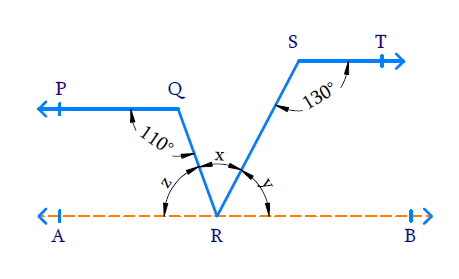# Ex.6.2 Q4 Lines and Angles Solution - NCERT Maths Class 9

## Question

In the given figure, if $$PQ || ST$$, $$\angle PQR = 110^ \circ$$ and $$\angle RST = 130^ {\circ}$$, find $$\angle QRS$$.Video Solution
Lines And Angles
Ex 6.2 | Question 4

## Text Solution

What is known?

$$PQ \| ST, \angle PQR = 110 ^ { \circ }$$ and $$\angle RST = 130 ^ { \circ }$$

What is unknown?

$$\angle QRS =?$$

Reasoning:

• Lines which are parallel to the same line are parallel to each other.
• When two parallel lines are cut a transversal, co-interior angles formed are supplementary.

Steps:

Draw a line $$AB$$ parallel to $$ST$$ through point $$R$$. Since $$AB\|ST$$ and $$PQ\|ST$$. So, $$AB\|PQ$$.

Let $$\angle SRQ = x,\;\; \angle SRB = y$$ and $$\angle QRA = z$$

Lines $$ST$$ and $$AB$$ are parallel with transversal $$SR$$ intersecting. Therefore, the co-interior angles are supplementary.

\begin{align} \angle RST + \angle SRB & = 180 ^ { \circ } \\ 130 ^ { \circ } + y & = 180 ^ { \circ } \\ y & = (180 ^ { \circ } - 130 ^ { \circ }) \\ & = 50 ^ { \circ } \\ \angle SRB = y & = 50 ^ { \circ } \end{align}

Similarly, lines $$PQ$$ and $$AB$$ are parallel with transversal $$QR$$ intersecting. Therefore, the co-interior angles are supplementary.

\begin{align} \angle PQR + \angle QRA & = 180 ^ { \circ } \\ 110 ^ { \circ } + z & = 180 ^ { \circ } \\ z & = (180 ^ { \circ } - 110 ^ { \circ }) \\ & = 70 ^ { \circ } \\ \angle QRA = z & = 70 ^ { \circ } \end{align}

$$AB$$ is a line, $$RQ$$ and $$RS$$ are rays on $$AB$$. Hence,

\begin{align} \angle QRA + \angle QRS + &\angle SRB = 180 ^ { \circ } \\ 70 ^ { \circ } + x + 50 ^ { \circ } & = 180 ^ { \circ } \\ 120 ^ { \circ } + x & = 180 ^ { \circ } \\ x & = (180 ^ { \circ } - 120 ^ { \circ }) \\ x & = 60 ^ { \circ } \\ \angle QRS = x & = 60 ^ { \circ } \end{align}

Video Solution
Lines And Angles
Ex 6.2 | Question 4
Learn from the best math teachers and top your exams

• Live one on one classroom and doubt clearing
• Practice worksheets in and after class for conceptual clarity
• Personalized curriculum to keep up with school